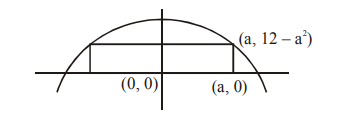# The maximum area (in sq. units) of a rectangle having`
Question:

The maximum area (in sq. units) of a rectangle having its base on the $\mathrm{x}$-axis and its other two vertices on the parabola, $y=12-x^{2}$ such that the rectangle lies inside the parabola, is :-

1. $20 \sqrt{2}$

2. $18 \sqrt{3}$

3. 32

4. 36

Correct Option: , 3

Solution:

$f(a)=2 a(12-a)^{2}$$f^{\prime}(a)=2\left(12-3 a^{2}\right)$

maximum at $\mathrm{a}=2$

maximum area $=\mathrm{f}(2)=32$